### Home > A2C > Chapter 4 > Lesson 4.1.2 > Problem4-21

4-21.
1. Solve for z in each equation below. Homework Help ✎

1. 4z =8

2. 42z/3 = 8(z+2)

3. 3z = 812

4. 5(z+1)/3 = 251/z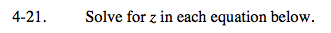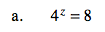Change to a common base.

$z=-\frac{18}{5}$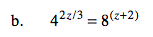See part (a).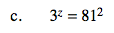See part (a).

z = 8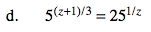See part (a).